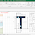## Tuesday, April 26, 2011

### How to plot a function using matplotlib

We will see how to evaluate a function using numpy and how to plot the result.
```import pylab
import numpy

x = numpy.linspace(-15,15,100) # 100 linearly spaced numbers
y = numpy.sin(x)/x # computing the values of sin(x)/x

# compose plot
pylab.plot(x,y) # sin(x)/x
pylab.plot(x,y,'co') # same function with cyan dots
pylab.plot(x,2*y,x,3*y) # 2*sin(x)/x and 3*sin(x)/x
pylab.show() # show the plot
```
The command pylab.show() will open a window with the following plot:

1.Great help thank you!

2.3.4.Very good info, thanks!!!

5.How to realize it without numpy?!

1.It's easy, you just need to generate the vectors x and y in another way. For example, x = [a*1.0 for a in range(0,10)] and y = [sin(xx) for xx in x].

6.Is it possible to plot a function and not just an equation?

7.8.How to plot something like the following equation?

(x+y−2)^2

1.Hi Divij, here's an example that can hep you: https://glowingpython.blogspot.co.uk/2012/01/how-to-plot-two-variable-functions-with.html

9.Thanks for the code, that helped a lot and gave some idea about what to use etc. But what I'm wondering is , do we really need to use only standart functions that have defined by Numpy? Or can we define a function ourselves and use it in numpy function too ?
Thanks anyways, good code

10.Great but it's easy...